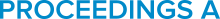# Supplementary material from "Cycle index polynomials and generalized quantum separability tests"

Posted on 2023-05-24 - 18:24
One family of pure bipartite states use extendibility as their means of determining separability of the state. Here, we derive an exact expression of the acceptance probability of such a test as the extension of the state increases. We prove that the analytical form of this expression is given by the cycle index polynomial of the symmetric group Sk, which is itself related to the Bell polynomials. After doing so, we derive a family of quantum separability tests, each of which is generated by a finite group; for all such algorithms, we show that the acceptance probability is determined by the cycle index polynomial of the group. Finally, we produce and analyse explicit circuit constructions for these tests, showing that the tests corresponding to the symmetric and cyclic groups can be executed with O(k2) and O(klog (k)) controlled-SWAP gates, respectively, where k is the number of copies of the state being tested.

## CITE THIS COLLECTION

or
Select your citation style and then place your mouse over the citation text to select it.

## Usage metrics## AUTHORS (3)